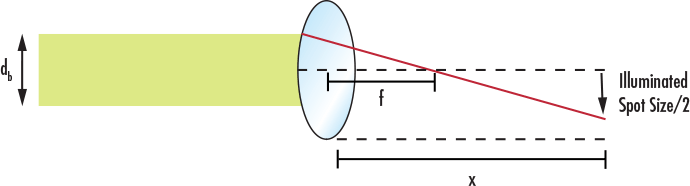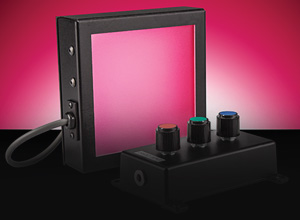# Illumination Spot Size Calculator

View All Technical Tools

db (mm):
f (mm):
x (mm):

Spot Size (mm) : --$$\text{when} \, f > 0 \, \, \text{and} \, \, x > f: \, \, \text{Spot Size} = \frac{d_b}{f} \times \left( x - f \right)$$
 Spot Size Represents the resulting geometrical spot size after the beam has exited the lens db Represents the diameter of the beam that enters the lens (units are in mm)
 f Represents the focal length of the lensxRepresents the propagation distance from the output side of the lens to the illuminated spot

Note: if f<0, be sure type in a negative sign into a calculator.

## Description

Determine the illuminated spot size resulting from a collimated light source passing through a singlet based on the input beam diameter, the lens focal length, and the propagation distance. Calculator assumes a thin lens and uses the paraxial approximation. The calculator works for three possible scenarios: f > 0 and the propagation distance > f, f > 0 and the propagation distance < f, and f < 0. The larger the difference between propagation distance and focal length the greater the spot size will be.

## Related Resources and Products### Optical Lenses